How to Search and Locate Worksheet in Excel

If there are multiple worksheets exist in excel, there will be a lot of worksheet tabs in tab bar. And it is difficult to find a worksheet we want. Though we can search worksheet by right click in worksheet bar to activate worksheet, a simple and convenient way will be better especially there are a lot of worksheets exist. This article will provide you a simple way to solve this problem by VBA.

Search and Locate Worksheet by VBA

Suppose there are multiple worksheets in this workbook. Worksheet names are squeezed in the tab bar. We want to find out a worksheet and open this worksheet quickly. We can edit VBA script to achieve our thought.

Step 1: On current visible worksheet, right click on sheet name tab to load Sheet management menu. Select View Code, Microsoft Visual Basic for Applications window pops up.

Or you can enter Microsoft Visual Basic for Applications window via Developer->Visual Basic.

Step 2: In Microsoft Visual Basic for Applications window, click Insert->Module, enter below code in Module1:

Sub SearchWorksheet()

Dim ShName As String

Dim ShFound As Boolean

ShName = InputBox("Enter worksheet name to find in this workbook:", "Search Worksheet")

If ShName = "" Then Exit Sub

On Error Resume Next

ActiveWorkbook.Sheets(ShName).Select

ShFound = (Err = 0)

On Error GoTo 0

If ShFound Then

MsgBox "Worksheet '" & ShName & "' has been found!"

Else

MsgBox "Worksheet '" & ShName & "' could not be found!"

End If

End Sub

Comment:

You can change the message in message box per your demands.

Step 3: Save the codes, see screenshot below. And then quit Microsoft Visual Basic for Applications.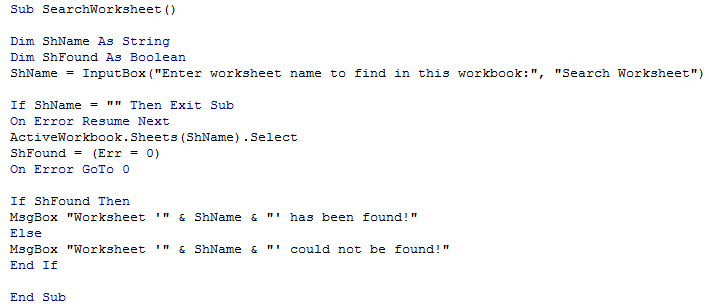Step 4: Click Developer->Macros to run Macro. Select ‘SearchWorksheet’ and click Run.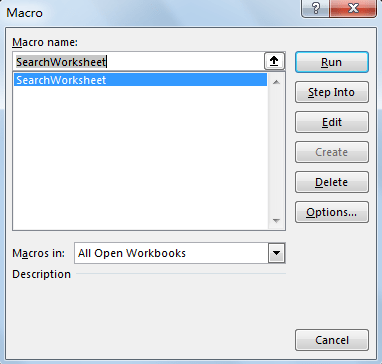Step 5: Search Worksheet dialog pops up. Enter worksheet name into the text box, then click OK. For example, enter Sheet2 which really exists.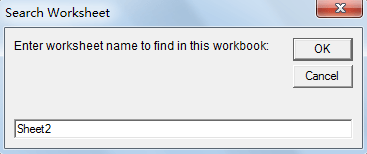Step 6: Verify that below message pops up. And Sheet2 is selected directly. User can do operations on Sheet2 directly.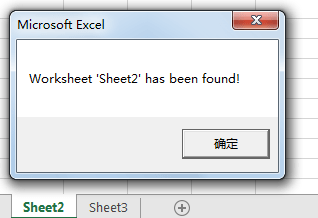Step 7: Repeat step#4, this time enter a worksheet doesn’t exist. Verify that below message pops up.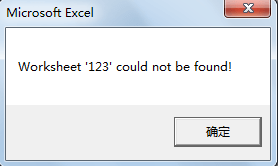Related Posts

VBA Macro For VLOOKUP From Another Sheet

In the previous post, you should know that how to fix or remove the #N/A error when using VLOOKUP formula to lookup value from another sheet. And this post will show you how to use VBA code to vlookup data ...

How To Insert Comments in Protected Worksheet in Excel

This post will show you how to allow comments in a protected worksheet in Excel. You can easily to insert comments into cells in a normal worksheet in Excel, but if want to insert a comment in a worksheet that ...

How To Convert Text to Upper Cases(Using VBA) in Excel

This post will show you how to switch from lower case to upper case in Excel. and I am going to show you two different ways of converting text to upper cases using formula or VBA macro in Excel 2013,Excel ...

How To Hide Every Other Row in Excel (Using VBA)

This post will show you how to hide alternate rows or columns in Excel or how to hide every third, fourth, fifth row or column in Excel. If you want to hide every other row in your current worksheet, how ...

How to Disable the Save As Prompt in Excel

This post will show you how to use a VBA Macro to save an Excel file and overwrite any existing file without a prompt so that you are going to get the little window that says file already exists do ...

How to Count Cells that Contain even or odd numbers in Excel

This post will guide you how to count the number of cells that contain odd or even numbers within a range of cells using a formula in Excel 2013/2016.How do I count cells that contain odd numbers through the use ...

How to Count Cells that Contain negative Numbers in Excel

This post will guide you how to count the number of cells that contain negative numbers within a range of cells using a formula in Excel 2013/2016.You can count the number of negative numbers in your data using easy functions ...

How to Count Cells Are Not Blank or Empty in Excel

This post will guide you how to count cells that are not blank or empty in a given range cells using a formula in Excel 2013/2016.How do I count the number of cells that are not blank in a particular ...

How to Count Cells Less Than a Specific Value in Excel

This post will guide you how to count the number of cells less than a particular numeric value in a given range cells using a formula in Excel 2013/2016. How do I count cells that are less than a specific ...

How to Count Cells Greater Than a Specific Value in Excel

This post will guide you how to count the number of cells greater than a particular numeric value in a given range cells using a formula in Excel 2013/2016. How do I count cells that are greater than a specific ...

Sidebar